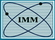ColESoComputational Aerodynamics & Aeroacoustics Laboratory Keldysh Institute of Applied Mathematics of RAS   4, Miusskaya Sq., Moscow, 125047, Russia, phone: (7 499) 2207218

# Collection of exact solutions (ColESo)Linear acoustics in 1D, 2D, 3D Linear acoustics with viscosity and heat conductivity in free space and in channels Diffraction of acoustic waves (by corner and by cylinder) Planar vortexes Low-Reynolds flows (shock structure, Couette flow) 1D discontinuous problems (Riemann problem, shock wave reflection)

# Solutions implemented

### Linearized Euler equations in free spacePlanar waves Initial pulse propagation in 2D and 3D Waves generated by sources in 1D, 2D and 3D Wave generated by moving point source in 3D Rotating point dipole Acoustic wave moving through a shock prescribed by background field (1D)

### Linearized Euler equations in presence of solid wallsSine wave in a cylinder or between coaxial cylinders Diffraction of initial pulse by a cylinder Diffraction of initial pulse by a corner 2*Pi/n (including half-line) Diffraction of planar wave by a corner 2*Pi/n (including half-line)

### Linearized Navier – Stokes equationsSine wave in a cube with periodic boundary conditions Sine wave in a planar channel Sine wave in a cylindrical channel Vortex in a circle with arbitrary initial profile (2D)

### Euler equationsSteady planar vortexes 1D Riemann problem 1D shock wave reflection by a wall 1D smooth simple wave (up to the blow-up time) Curl-free flows around a cylinder and a sphere (low-speed incompressible limit)

### Navier – Stokes equationsShock wave profile: non-heat-conductive case and Pr = 3/4 case (1D) Couette flow between parallel plates: μ = const case, μ = μ0 * T case, and μ = μ0 * T-1/2 case Flow between coaxial cylinders (2D) Low-speed flow around a sphere (incompressible case, 3D)

# Reliability and accuracy

 The solutions are verified: by comparison with the numerical solutions obtained using Noisette code; by checking that they locally satisfy the corresponding equation where derivatives are replaced by finite differences. However, there are several sources of inaccuracies. Quadrature formulas for integrals. In some cases the number of nodes is automatically chosen big enough to guarantee machine precision (in exact arithmetic). In other cases the user should adjust the number of quadrature nodes. Truncating series. s_Cylinder (diffraction of Gaussian pulse by cylinder) case requires a user-adjusted number of terms in the series. Special functions approximation. This problem arises for s_Cylinder and partially for s_Coaxial where Bessel functions are used. However, this causes no troubles for s_Gaussian2D and s_ViscAcCylinder where Bessel functions are also in use. Accumulating of arithmetic errors. I tried to avoid this if possible. Bugs in the solution and its program implementation. Unfortunately, possible everywhere.

# Details of the implementation

 Language: C++03 All solutions are presented as classes inheriting the abstract class tPointFunction Special thanks for the special functions to S. Moshier (Cephes and GNU LibQuadMath) and to J. Burkardt for the Jacobi quadrature rules Partially based on template classes for compatibility with extended precision arithmetic (using QD package) Object-free interface for use in C or FORTRAN (double precision only)Main page  |   Contacts Web-design: Cherepock, dualks